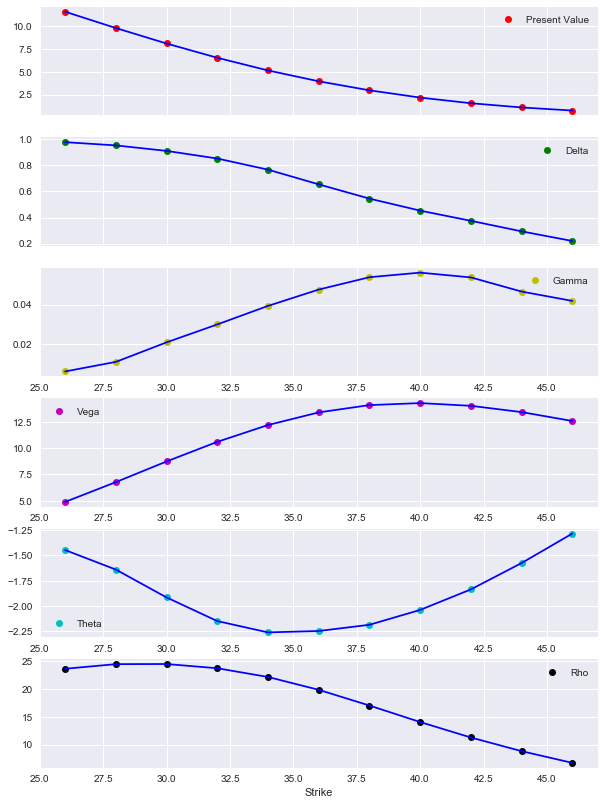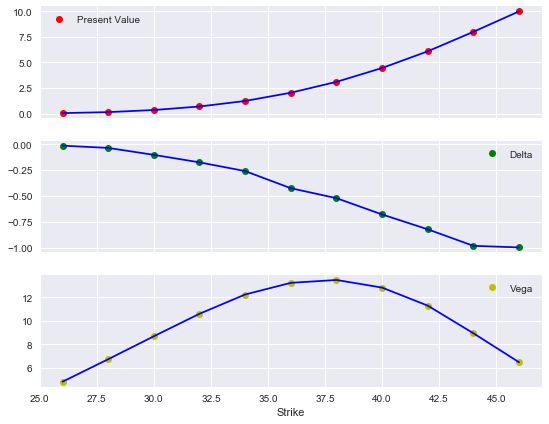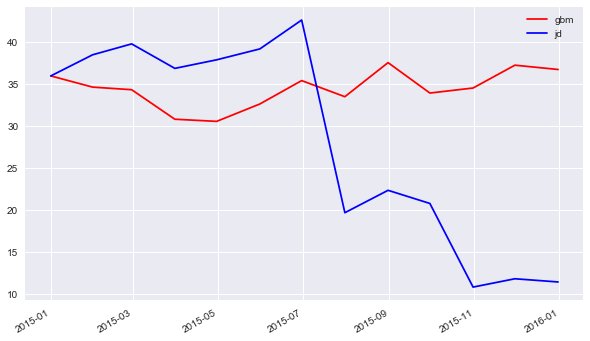# 4. Single-Risk Derivatives Valuation¶

This part introduces into the modeling and valuation of derivatives instruments (contingent claims) based on a single risk factor (e.g. a stock price, stock index level or interest rate). It also shows how to model and value portfolios composed of such instruments.

:

from dx import *
from pylab import plt
plt.style.use('seaborn')


The following single risk factor valuation classes are available:

• valuation_mcs_european_single for derivatives with European exercise
• valuation_mcs_american_single for derivatives with American/Bermudan exercise

## 4.1. Modeling the Risk Factor¶

Before moving on to the valuation classes, we need to model an instantiate an underlying risk factor, in this case a geometric_brownian_motion object. Background information is provided in the respective part of the documentation about model classes.

:

r = constant_short_rate('r', 0.06)

:

me = market_environment('me', dt.datetime(2015, 1, 1))

:

me.add_constant('initial_value', 36.)

:

me.add_curve('discount_curve', r)

:

gbm = geometric_brownian_motion('gbm', me)


## 4.2. valuation_mcs_european_single¶

The first instrument we value is a European call option written on the single relevant risk factor as embodied by the gbm model object. To this end, we add a maturity date to the market environment and a strike price.

:

me.add_constant('maturity', dt.datetime(2015, 12, 31))


To instantiate a the valuation_mcs_european_single class, the following information/data is to be provided:

• name as a string object
• instance of a model class
• market environment
• payoff of the instrument a string object and containing “regular” Python/NumPy code
:

call_eur = valuation_mcs_european_single(
name='call_eur',
underlying=gbm,
mar_env=me,
payoff_func='np.maximum(maturity_value - strike, 0)')


In this case, the payoff is that of a regular, plain vanilla European call option. If $$T$$ is the maturity date, $$S_T$$ the value of the relevant risk factor at that date and $$K$$ the strike price, the payoff $$h_T$$ at maturity of such an option is given by

$h_T = \max[S_T - K, 0]$

maturity_value represents the value vector of the risk factor at maturity. Any other “sensible” payoff definition is possible. For instance, the following works as well:

:

payoff = 'np.maximum(np.minimum(maturity_value) * 2 - 50, 0)'


Other standardized payoff elemenets include mean_value, max_value and min_value representing maturity value vectors with the pathwise means, maxima and minima. Using these payoff elements allows the easy definition of options with Asian features.

Having instantiated the valuation class, the present_value method returns the present value Monte Carlo estimator for the call option.

:

call_eur.present_value()

:

2.172619


Similarly, the delta and vega methods return the delta and the vega of the option, estimated numerically by a forward difference scheme and Monte Carlo simulation.

:

call_eur.delta()

:

0.4491

:

call_eur.vega()

:

14.1882


This approach allows to work with such a valuation object similar to an analytical valuation formula like the one of Black-Scholes-Merton (1973). For example, you can estimate and plot present values, deltas, gammas, vegas, thetas and rhos for a range of different initial values of the risk factor.

:

%%time
k_list = np.arange(26., 46.1, 2)
pv = []; de = []; ve = []; th = []; rh = []; ga = []
for k in k_list:
call_eur.update(strike=k)
pv.append(call_eur.present_value())
de.append(call_eur.delta(0.5))
ve.append(call_eur.vega(0.2))
th.append(call_eur.theta())
rh.append(call_eur.rho())
ga.append(call_eur.gamma())

CPU times: user 6.64 s, sys: 954 ms, total: 7.59 s
Wall time: 7.66 s

:

%matplotlib inline


There is a little plot helper function available to plot these statistics conveniently.

:

plot_option_stats_full(k_list, pv, de, ga, ve, th, rh)## 4.3. valuation_mcs_american_single¶

The modeling and valuation of derivatives with American/Bermudan exercise is almost completely the same as in the more simple case of European exercise.

:

me.add_constant('initial_value', 36.)
# reset initial_value

:

put_ame = valuation_mcs_american_single(
name='put_eur',
underlying=gbm,
mar_env=me,
payoff_func='np.maximum(strike - instrument_values, 0)')


The only difference to consider here is that for American options where exercise can take place at any time before maturity, the inner value of the option (payoff of immediate exercise) is relevant over the whole set of dates. Therefore, maturity_value needs to be replaced by instrument_values in the definition of the payoff function.

:

put_ame.present_value()

:

4.452


Since DX Analytics relies on Monte Carlo simulation and other numerical methods, the calculation of the delta and vega of such an option is identical to the European exercise case.

:

put_ame.delta()

:

-0.6655

:

put_ame.vega()

:

10.3122

:

%%time
k_list = np.arange(26., 46.1, 2.)
pv = []; de = []; ve = []
for k in k_list:
put_ame.update(strike=k)
pv.append(put_ame.present_value())
de.append(put_ame.delta(.5))
ve.append(put_ame.vega(0.2))

CPU times: user 15.5 s, sys: 957 ms, total: 16.5 s
Wall time: 16.1 s

:

plot_option_stats(k_list, pv, de, ve)## 4.4. Portfolio Valuation¶

In general, market players (asset managers, investment banks, hedge funds, insurance companies, etc.) have to value not only single derivatvies instruments but rather portfolios composed of several derivatives instruments. A consistent derivatives portfolio valuation is particularly important when there are multiple derivatives written on the same risk factor and/or correlations between different risk factors.

These are the classes availble for a consistent portfolio valuation:

• derivatives_position to model a portfolio position
• derivatives_portfolio to model a derivatives portfolio

### 4.4.1. derivatives_position¶

We work with the market_environment object from before and add information about the risk factor model we are using.

:

me.add_constant('model', 'gbm')


A derivatives position consists of “data only” and not instantiated model or valuation objects. The necessary model and valuation objects are instantiated during the portfolio valuation.

:

put = derivatives_position(
name='put',  # name of position
quantity=1,  # number of instruments
underlyings=['gbm'],  # relevant risk factors
mar_env=me,  # market environment
otype='American single',  # the option type
payoff_func='np.maximum(40. - instrument_values, 0)')
# the payoff funtion


The method get_info prints an overview of the all relevant information stored for the respective derivatives_position object.

:

put.get_info()

NAME
put

QUANTITY
1

UNDERLYINGS
['gbm']

MARKET ENVIRONMENT

**Constants**
initial_value 36.0
volatility 0.2
final_date 2015-12-31 00:00:00
currency EUR
frequency W
paths 25000
maturity 2015-12-31 00:00:00
strike 40.0
model gbm

**Lists**

**Curves**
discount_curve <dx.frame.constant_short_rate object at 0x106799978>

OPTION TYPE
American single

PAYOFF FUNCTION
np.maximum(40. - instrument_values, 0)


### 4.4.2. derivatives_portfolio¶

The derivatives_portfolio class implements the core portfolio valuation tasks. This sub-section illustrates to cases, one with uncorrelated underlyings and another one with correlated underlyings

#### 4.4.2.1. Uncorrelated Underlyings¶

The first example is based on a portfolio with two single-risk factor instruments on two different risk factors which are not correlated. In addition to the gbm object, we define a jump_diffusion object.

:

me_jump = market_environment('me_jump', dt.datetime(2015, 1, 1))

:

me_jump.add_environment(me)


Based on this new risk factor model object, a European call option is defined.

:

call_jump = derivatives_position(
name='call_jump',
quantity=3,
underlyings=['jd'],
mar_env=me_jump,
otype='European single',
payoff_func='np.maximum(maturity_value - 36., 0)')


Our relevant market now takes on the following form (defined a dictionary objects):

:

risk_factors = {'gbm': me, 'jd' : me_jump}
positions = {'put' : put, 'call_jump' : call_jump}


To instantiate the derivatives_portfolio class, a valuation environment (instance of market_environment class) is needed.

:

val_env = market_environment('general', dt.datetime(2015, 1, 1))


For the instantiation, we pass all the elements to the portfolio class.

:

port = derivatives_portfolio(
name='portfolio',  # name
positions=positions,  # derivatives positions
val_env=val_env,  # valuation environment
risk_factors=risk_factors, # relevant risk factors
correlations=False,  # correlation between risk factors
fixed_seed=False,  # fixed seed for randon number generation
parallel=False)  # parallel valuation of portfolio positions


Once instantiated, the method get_statistics provides major portfolio statistics like position values, position deltas ans position vegas.

:

%%time
stats = port.get_statistics()

Totals
pos_value    34.7260
pos_delta     2.2706
pos_vega     22.1461
dtype: float64
CPU times: user 838 ms, sys: 64.4 ms, total: 902 ms
Wall time: 885 ms

:

stats

:

position name quantity otype risk_facts value currency pos_value pos_delta pos_vega
0 put put 1 American single [gbm] 4.432 EUR 4.432 -0.6571 10.9231
1 call_jump call_jump 3 European single [jd] 10.098 EUR 30.294 2.9277 11.2230

The method returns a standard pandas DataFrame object with which you can work as you are used to.

:

stats[['pos_value', 'pos_delta', 'pos_vega']].sum()

:

pos_value    34.7260
pos_delta     2.2706
pos_vega     22.1461
dtype: float64


The metod get_values only calculates the present values of the derivatives instruments and positions and is therefore a bit less compute and time intensive.

:

%time port.get_values()

Total
pos_value    34.438908
dtype: float64
CPU times: user 191 ms, sys: 14.3 ms, total: 206 ms
Wall time: 199 ms

:

position name quantity otype risk_facts value currency pos_value
0 put put 1 American single [gbm] 4.449000 EUR 4.449000
1 call_jump call_jump 3 European single [jd] 9.996636 EUR 29.989908

The method get_positions provides detailed information about the single derivatives positions of the derivatives_portfolio object.

:

port.get_positions()


--------------------------------------------------
NAME
put

QUANTITY
1

UNDERLYINGS
['gbm']

MARKET ENVIRONMENT

**Constants**
initial_value 36.0
volatility 0.2
final_date 2015-12-31 00:00:00
currency EUR
frequency M
paths 50000
maturity 2015-12-31 00:00:00
strike 40.0
model gbm
starting_date 2015-01-01 00:00:00

**Lists**
time_grid [datetime.datetime(2015, 1, 1, 0, 0) datetime.datetime(2015, 1, 31, 0, 0)
datetime.datetime(2015, 2, 28, 0, 0) datetime.datetime(2015, 3, 31, 0, 0)
datetime.datetime(2015, 4, 30, 0, 0) datetime.datetime(2015, 5, 31, 0, 0)
datetime.datetime(2015, 6, 30, 0, 0) datetime.datetime(2015, 7, 31, 0, 0)
datetime.datetime(2015, 8, 31, 0, 0) datetime.datetime(2015, 9, 30, 0, 0)
datetime.datetime(2015, 10, 31, 0, 0)
datetime.datetime(2015, 11, 30, 0, 0)
datetime.datetime(2015, 12, 31, 0, 0)]
correlation_matrix      gbm   jd
gbm  1.0  0.0
jd   0.0  1.0
cholesky_matrix [[1. 0.]
[0. 1.]]
random_numbers [[[ 1.59911088 -1.4046112  -1.41690211 ... -0.02367133  0.64452581
0.83372797]
[-1.71068746 -0.17718321  1.19908444 ... -0.12887556  1.84851716
1.99223491]
[ 0.91793265  0.527942   -0.51603028 ...  0.84100797 -1.36662164
-0.60581475]
...
[ 1.2128477  -0.02445883  0.28700922 ... -0.68947286 -0.04293013
-0.15945594]
[-1.32537389 -0.70003276  1.90365643 ... -0.2332303  -0.0369755
1.44280306]
[ 0.20542848 -0.7790572  -0.75625624 ... -1.470969   -1.00645074
-0.12511486]]

[[-2.1046119   1.11433037 -1.44243622 ...  0.95216647 -0.4916233
0.14714287]
[ 0.88624348 -0.10835483  1.09678341 ... -0.02139183  1.17828289
-0.6156402 ]
[-0.49969036 -1.61494765 -0.6953123  ...  0.09100147  1.12869001
-0.65676166]
...
[ 0.04256569  0.51287869  0.39970509 ...  2.38658577  1.64993773
-0.16761589]
[ 0.69762962  0.89591537  0.14343585 ... -0.62170926  0.95533176
-0.03614675]
[-0.88760744 -1.24120179  2.66507407 ...  0.40644798 -1.64541865
1.36340621]]]
rn_set {'jd': 1, 'gbm': 0}

**Curves**
discount_curve <dx.frame.constant_short_rate object at 0x106799978>

OPTION TYPE
American single

PAYOFF FUNCTION
np.maximum(40. - instrument_values, 0)

--------------------------------------------------

--------------------------------------------------
NAME
call_jump

QUANTITY
3

UNDERLYINGS
['jd']

MARKET ENVIRONMENT

**Constants**
initial_value 36.0
volatility 0.2
final_date 2015-12-31 00:00:00
currency EUR
frequency M
paths 50000
maturity 2015-12-31 00:00:00
strike 40.0
model jd
lambda 0.8
mu -0.8
delta 0.1
starting_date 2015-01-01 00:00:00

**Lists**
time_grid [datetime.datetime(2015, 1, 1, 0, 0) datetime.datetime(2015, 1, 31, 0, 0)
datetime.datetime(2015, 2, 28, 0, 0) datetime.datetime(2015, 3, 31, 0, 0)
datetime.datetime(2015, 4, 30, 0, 0) datetime.datetime(2015, 5, 31, 0, 0)
datetime.datetime(2015, 6, 30, 0, 0) datetime.datetime(2015, 7, 31, 0, 0)
datetime.datetime(2015, 8, 31, 0, 0) datetime.datetime(2015, 9, 30, 0, 0)
datetime.datetime(2015, 10, 31, 0, 0)
datetime.datetime(2015, 11, 30, 0, 0)
datetime.datetime(2015, 12, 31, 0, 0)]
correlation_matrix      gbm   jd
gbm  1.0  0.0
jd   0.0  1.0
cholesky_matrix [[1. 0.]
[0. 1.]]
random_numbers [[[ 1.59911088 -1.4046112  -1.41690211 ... -0.02367133  0.64452581
0.83372797]
[-1.71068746 -0.17718321  1.19908444 ... -0.12887556  1.84851716
1.99223491]
[ 0.91793265  0.527942   -0.51603028 ...  0.84100797 -1.36662164
-0.60581475]
...
[ 1.2128477  -0.02445883  0.28700922 ... -0.68947286 -0.04293013
-0.15945594]
[-1.32537389 -0.70003276  1.90365643 ... -0.2332303  -0.0369755
1.44280306]
[ 0.20542848 -0.7790572  -0.75625624 ... -1.470969   -1.00645074
-0.12511486]]

[[-2.1046119   1.11433037 -1.44243622 ...  0.95216647 -0.4916233
0.14714287]
[ 0.88624348 -0.10835483  1.09678341 ... -0.02139183  1.17828289
-0.6156402 ]
[-0.49969036 -1.61494765 -0.6953123  ...  0.09100147  1.12869001
-0.65676166]
...
[ 0.04256569  0.51287869  0.39970509 ...  2.38658577  1.64993773
-0.16761589]
[ 0.69762962  0.89591537  0.14343585 ... -0.62170926  0.95533176
-0.03614675]
[-0.88760744 -1.24120179  2.66507407 ...  0.40644798 -1.64541865
1.36340621]]]
rn_set {'jd': 1, 'gbm': 0}

**Curves**
discount_curve <dx.frame.constant_short_rate object at 0x106799978>

OPTION TYPE
European single

PAYOFF FUNCTION
np.maximum(maturity_value - 36., 0)

--------------------------------------------------


#### 4.4.2.2. Correlated Underlyings¶

The second example case is exactly the same but now with a highly positive correlation between the two relevant risk factors. Correlations are to be provided as a list of list objects using the risk factor model names to reference them.

:

correlations = [['gbm', 'jd', 0.9]]


Except from now passing this new object, the application and usage remains the same.

:

port = derivatives_portfolio(
name='portfolio',
positions=positions,
val_env=val_env,
risk_factors=risk_factors,
correlations=correlations,
fixed_seed=True,
parallel=False)

:

port.get_statistics()

Totals
pos_value    35.1350
pos_delta     1.6345
pos_vega     21.8606
dtype: float64

:

position name quantity otype risk_facts value currency pos_value pos_delta pos_vega
0 put put 1 American single [gbm] 4.433 EUR 4.433 -0.6548 10.8335
1 call_jump call_jump 3 European single [jd] 10.234 EUR 30.702 2.2893 11.0271

The Cholesky matrix has been added to the valuation environment (which gets passed to the risk factor model objects).

:

port.val_env.lists['cholesky_matrix']

:

array([[1.        , 0.        ],
[0.9       , 0.43588989]])


Let us pick two specific simulated paths, one for each risk factor, and let us visualize these.

:

path_no = 0
paths1 = port.underlying_objects['gbm'].get_instrument_values()[:, path_no]
paths2 = port.underlying_objects['jd'].get_instrument_values()[:, path_no]


The plot illustrates that the two paths are indeed highly positively correlated. However, in this case a large jump occurs for the jump_diffusion object.

:

plt.figure(figsize=(10, 6))
plt.plot(port.time_grid, paths1, 'r', label='gbm')
plt.plot(port.time_grid, paths2, 'b', label='jd')
plt.gcf().autofmt_xdate()
plt.legend(loc=0); plt.grid(True)
# highly correlated underlyings
# -- with a large jump for one risk factor© Dr. Yves J. Hilpisch | The Python Quants GmbH